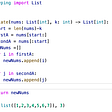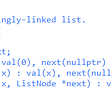# Leetcode

39. Combination Sum

Given an array of distinct integers `candidates` and a target integer `target`, return a list of all unique combinations of `candidates` where the chosen numbers sum to `target`. You may return the combinations in any order.

The same number may be chosen from `candidates` an unlimited number of times. Two combinations are unique if the frequency of at least one of the chosen numbers is different.

It is guaranteed that the number of unique combinations that sum up to `target` is less than `150` combinations for the given input.

Example 1:

`Input: candidates = [2,3,6,7], target = 7Output: [[2,2,3],]Explanation:2 and 3 are candidates, and 2 + 2 + 3 = 7. Note that 2 can be used multiple times.7 is a candidate, and 7 = 7.These are the only two combinations.`

Example 2:

`Input: candidates = [2,3,5], target = 8Output: [[2,2,2,2],[2,3,3],[3,5]]`

Example 3:

`Input: candidates = , target = 1Output: []`

Example 4:

`Input: candidates = , target = 1Output: []`

Example 5:

`Input: candidates = , target = 2Output: [[1,1]]`

Constraints:

• `1 <= candidates.length <= 30`
• `1 <= candidates[i] <= 200`
• All elements of `candidates` are distinct.
• `1 <= target <= 500`

Solution(Python3):

`class Solution:    def combinationSum(self, candidates, target):        ret = []        self.dfs(candidates, target, [], ret)        return ret    def dfs(self, nums, target, path, ret):        if target < 0:            return        if target == 0:            ret.append(path)            return        for i in range(len(nums)):            self.dfs(nums[i:], target - nums[i], path+[nums[i]], ret)# TC: O(N^(T/M)+1), where N is the number of candidates, T is the target value, and M is the minimum value among the candidates# SC: O(T/M)`

--

--

--

## More from JEN-LI CHEN IN DATA SCIENCE

My homepage to record my thought processes for solving SQL and Algorithm questions

## Fast Key-Value Store With PostgreSQL## Palindrome in Python: Check Palindrome Number, What is Palindrome and Examples## BetaMars Second Closed Beta Test Recruitment is Here!## STORY TIME## 5 Productive SDL Plugins To Improve Your Performance## How to Create a Symbolic link to a Directory in Linux? — CodeFather## I have one month to make an MMO: Day 3## Jen-Li Chen

In love with telling stories with data

## All Possible Full Binary Trees Solution | LeetCode-894: Medium | JavaScript Implementation## Solving with Intuition#1Rotate Array## Linked List | LeetCode Top Interview Questions